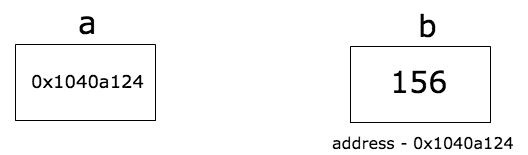go语言学习总结 原

盼望明天

go语言是直接将源码编译成二进制机器码的语言；它支持面向对象、也支持函数式编程；go是强类型语言；Go 编程语言原生支持并发。Go 使用 Go 协程（Goroutine） 和信道（Channel）来处理并发。

1、切片与数组差别：切片是动态化数组，切片是注意两者初始化和函数的区别

a.初始化差别

a:=[...] int {1,2,3} ; a:= int {1,2,3}

a:=[] int {1,2,3}; a:=make([]int,3); a:=make([] int ,3,5)

b.函数传递：

func changeArray(a int) {//数组
a = 100
}
func changeSlice(s []int) {//切片
s = 100
}

func main(){

a := [...]int{1, 2, 3}
changeArray(a)
fmt.Println(a) //值传递，输出结果：1

var s []int = []int{1, 2, 3, 4}
fmt.Println(len(s), cap((s)))
s = append(s, 6, 7, 8)
fmt.Println(len(s), cap(s))
changeSlice(s)
fmt.Println(s) //地址传递，输出结果：100

}

c.切片和垃圾回收

var digitRegexp = regexp.MustCompile("[0-9]+")

func FindDigits(filename string) []byte {
b, _ := ioutil.ReadFile(filename)
return digitRegexp.Find(b)
}

func FindDigits(filename string) []byte {
b, _ := ioutil.ReadFile(filename)
b = digitRegexp.Find(b)
c := make([]byte, len(b))
copy(c, b)
return c
}

2.数组和Map,数组的输出按照数组的放置顺序，但是Map是无序输出的，每次遍历的顺序不一样；

var m = map[string]int{
"unix":         0,
"python":       1,
"go":           2,
"javascript":   3,
"testing":      4,
"philosophy":   5,
"startups":     6,
"productivity": 7,
"hn":           8,
"reddit":       9,
"C++":          10,
}
var keys []string
for k := range m {
keys = append(keys, k)
}
sort.Strings(keys)
for _, k := range keys {
fmt.Println("Key:", k, "Value:", m[k])
}

3.go语言中的指针&b  获取b的内存地址值；*a 则是获取a值对应物理地址值的b值：156；

func change(val *int) {
*val = 55
}
func main() {
a := 58
fmt.Println("value of a before function call is",a)
b := &a
change(b)
fmt.Println("value of a after function call is", a)
}

4.那么什么时候使用指针接收器，什么时候使用值接收器？

/*

*/
func (e Employee) changeName(newName string) {
e.name = newName
}

/*

*/
func (e *Employee) changeAge(newAge int) {
e.age = newAge
}

5.在方法中使用值接收器 与 在函数中使用值参数

package main
import (
"fmt"
)
type rectangle struct {
length int
width  int
}
func area(r rectangle) {
fmt.Printf("Area Function result: %d\n", (r.length * r.width))
}
func (r rectangle) area() {
fmt.Printf("Area Method result: %d\n", (r.length * r.width))
}
func main() {
r := rectangle{
length: 10,
width:  5,
}
area(r)
r.area()

p := &r
/*
compilation error, cannot use p (type *rectangle) as type rectangle
in argument to area
*/
//area(p)
p.area()//通过指针调用值接收器
}

6.在方法中使用指针接收器 与 在函数中使用指针参数

package main
import (
"fmt"
)
type rectangle struct {
length int
width  int
}
func perimeter(r *rectangle) {
fmt.Println("perimeter function output:", 2*(r.length+r.width))

}
func (r *rectangle) perimeter() {
fmt.Println("perimeter method output:", 2*(r.length+r.width))
}
func main() {
r := rectangle{
length: 10,
width:  5,
}
p := &r //pointer to r
perimeter(p)
p.perimeter()
/*
cannot use r (type rectangle) as type *rectangle in argument to perimeter
*/
//perimeter(r)
r.perimeter()//使用值来调用指针接收器
}

7.并行和并发

8.线程与协程区别

9.缓冲信道和非缓冲信道

go系列教程：https://studygolang.com/subject/2

盼望明天

NLP 领域还有 5 大开放问题

09/21
0
0
R语言学习历程回顾总结

2017/11/27
0
0

【IT168 评论】几周前，做为8th Light公司的实习生，我从CEO那里拿到了我的工作成绩报告。在与CEO的会见期间，我发现了一个对如何做一个程序员的认识上的盲区。起初我以为，想扩展自己的软件...

2013/03/25
0
0

R语言是由 Ross Ihaka、Robert Gentleman二位创建的，这也许可以解释为什么叫R语言。现在由“R开发核心团队”负责开发。R是基于S语言的一个GNU项目。 一、R语言介绍 R是为统计计算和作图的一...

2015/11/30
119
0
R语言中文社区10篇最火的文章 | 2017大盘点

kmd8d5r
2017/12/30
0
0

【jQuery基础学习】05 jQuery与Ajax以及序列化

16分钟前
8
0
CVE-2019-14287（Linux sudo 漏洞）分析

17分钟前
4
0

17分钟前
5
0

23分钟前
11
0

ApacheCN_飞龙
23分钟前
2
0